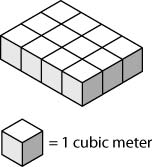How many cubes are added to complete the prism?

The base area is 4×3=12 sq m. The volume is 6 times this=72 cubic m. You stack to add 5 more layers. Each layer contains 12 cubes, so you add 60 to 12 from the bottom layer making 72=4×3×6. You need 72 cubes, so the total volume is 72 cubic m. You need 72 cubes altogether, so you need 60 more than shown.

by Top Rated User (613k points)